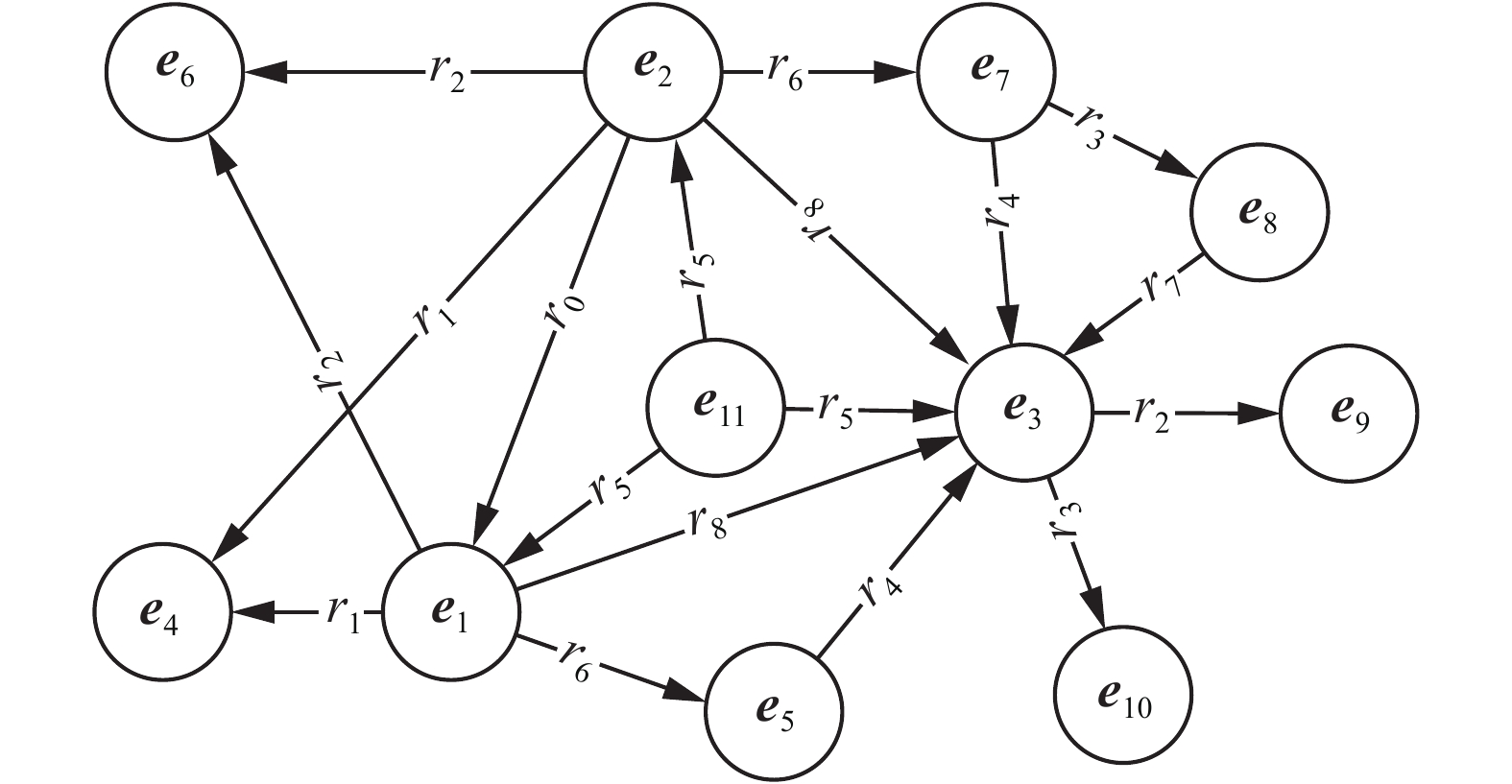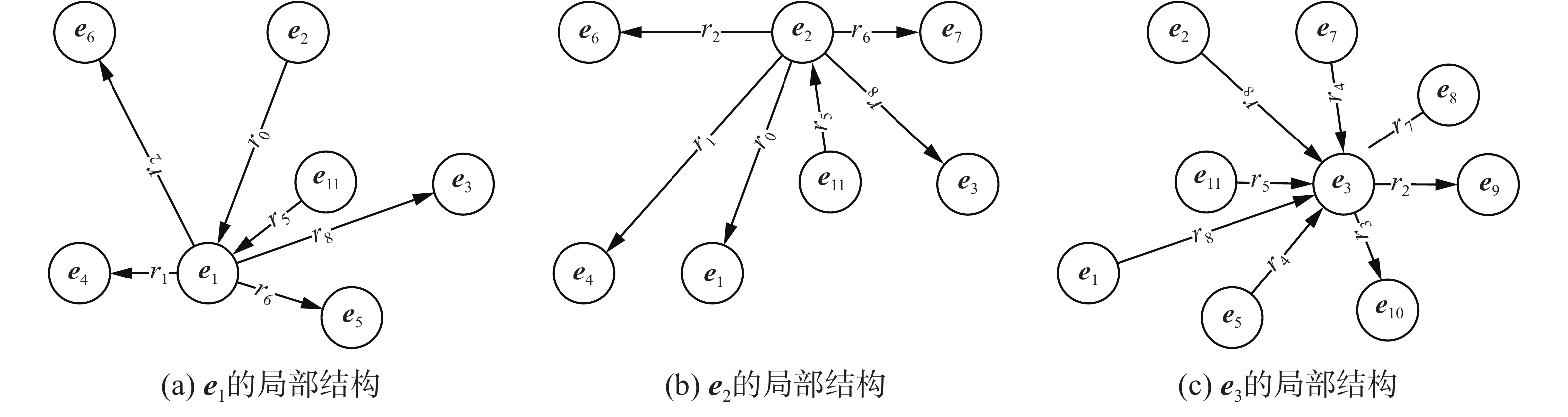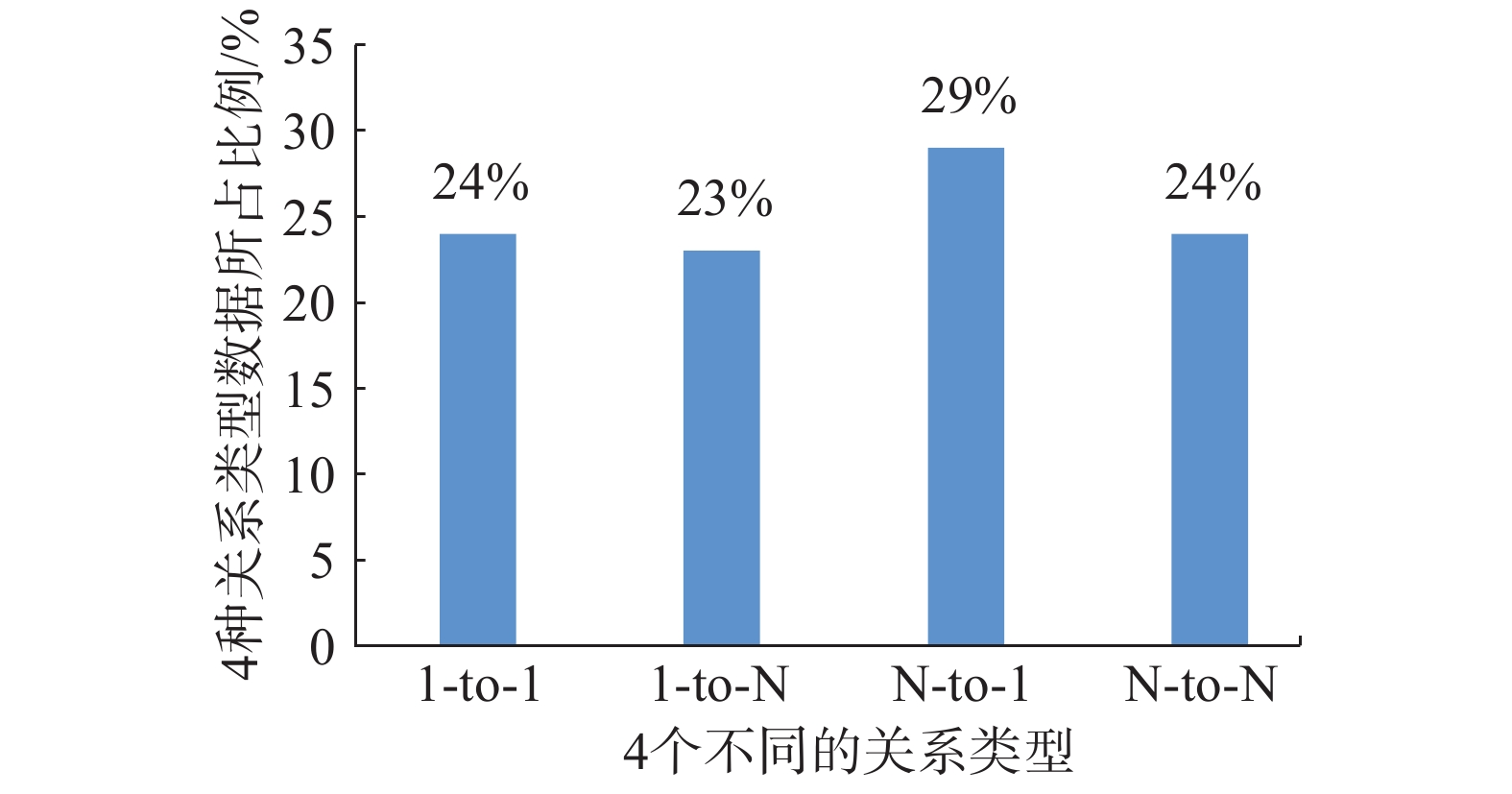﻿ 基于相似性负采样的知识图谱嵌入
«上一篇文章快速检索 高级检索

 智能系统学报2020, Vol. 15Issue (2): 218-226  DOI: 10.11992/tis.2018110220

### 引用本文RAO Guanjun, GU Tianlong, CHANG Liang, et al. Knowledge graph embedding based on similarity negative sampling[J]. CAAI Transactions on Intelligent Systems, 2020, 15(2): 218-226. DOI: 10.11992/tis.201811022.### 文章历史

Knowledge graph embedding based on similarity negative sampling
RAO Guanjun , GU Tianlong , CHANG Liang , BIN Chenzhong, QIN Saige , XUAN Wen
Guangxi Key Laboratory of Trusted Software, Guilin University of Electronic Technology, Guilin 541004, China
Abstract: For the existing knowledge graph embedding model, the random extraction of an entity from the entity set results in the generation of lower-quality negative triples, and this affects the feature learning ability of the entity and the relationship. In this paper, we study the related factors affecting the quality of negative triples, and propose an entity similarity negative sampling method to generate high-quality negative triples. In the similarity negative sampling method, all entities are first divided into a number of groups using the K-means clustering algorithm. Then, corresponding to each positive triple, an entity is selected to replace the head entity from the cluster, whereby the head entity is located in the positive triple, and the tail entity is replaced in a similar approach. TransE-SNS is obtained by combining the similarity negative sampling method with TransE. Experimental results show that TransE-SNS has made significant progress in link prediction and triplet classification tasks.
Key words: knowledge graph    representation learning    random sampling    similarity sampling    K-means clustering    stochastic gradient descent    link prediction    triple classification

1 相关研究

2 基于相似性负采样的知识图谱嵌入 2.1 实体的相似性 2.1.1 实体局部结构的相似性Download: 图 1 知识图谱 Fig. 1 Knowledge graphDownload: 图 2 实体的局部结构 Fig. 2 Local structure of the entity
2.1.2 实体向量的相似性

2.2 随机抽样的局限性

2.3 相似性负采样

 $\arg \min \sum\limits_{i = 1}^K {\sum\limits_{e \in {C_i}} {{{\left\| {{{e}} - {{{c}}_i}} \right\|}_{{L_2}}}}}$ (1)

2.4 TransE-SNS模型

TransE-SNS采用 ${{h}} + {{r}} \approx {{t}}$ 的翻译原则将实体和关系嵌入同一个向量空间。因此为TransE-SNS定义了得分函数：

 ${f_r}( h, t) = {\left\| {{{h}} + {{r}} - {{t}}} \right\|_{{L_2}}}$ (2)

 $L = \sum\limits_{({ h},{ r},{ t})} \in S {\sum\limits_{{\rm Ne}{g_{({ h},{ r},{ t})}}} \in { S}'} {\nabla {{[{f_r}({ h},{ t}) + \gamma - {f_r}({ h}',{ t}')]}_ +}}$ (3)

1)初始化：

2) ${{r}}$ ← uniform ( ${{- 6} / {\sqrt {{n}}}}$ , ${6 / {\sqrt {{n}}}}$ )对于每一个rR

3) ${{r}}$ ${{r}}/||{{r}}||$ 对于每一个rR

4) ${{e}}$ ← uniform ( ${{- 6} / {\sqrt {{n}}}}$ , ${6 /{\sqrt {{n}}}}$ )对于每一个eE

4) ${{e}}$ ${{e}}/||{{e}}||$ 对于每一个eE

5) loop

6) Sbatch ← sample (S, b) //从S中抽取一个大小为b的mini-batch

7)Tbatch← Ø // 初始化正负例三元组对的集合

8) for (h, r, t)∈Sbatch do

9) Neg(h,r,t) ← sample(S'(h, r, t)) //抽取一个负例三元组(h' , r, t)或(h, r, t' )

10) TbatchTbatch∪{(h, r, t), Neg(h, r, t)}

11) end for

12) 更新实体向量与关系向量

$\displaystyle\sum\limits_{({ h},{ r},{ t}) \in { S}} {\displaystyle\sum\limits_{{\rm Ne}{{\rm g}_{({ h},{ r},{ t})}} \in { S}'} {\nabla {{[{f_{ r}}({ h},{ t}) + \gamma - {f_{ r}}({ h}',{ t}')]}_ +}}}$

13) if epoch % 50 == 0 then

14) 更新Ei,// K-Means聚类

15) end if

16) end loop

3 实验与分析

3.1 数据设置表 1 实验数据集 Tab.1 Experimental datasets
3.2 链接预测表 2 链接预测中的最佳参数设置 Tab.2 Optimal parameter setting in link prediction表 3 链接预测结果 Tab.3 Link prediction resultsDownload: 图 3 FB15K中1345个关系的类型分布 Fig. 3 In the FB15K, the category distribution of 1345 relations